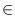Next: Hashing Arrays ( h_array Up: Dictionary Types Previous: Dictionaries ( dictionary )   Contents   Index

# Dictionary Arrays ( d_array )

Definition

An instance A of the parameterized data type d_array<I,E> (dictionary array) is an injective mapping from the linearly ordered data type I, called the index type of A, to the set of variables of data type E, called the element type of A. We use A(i) to denote the variable with index i and we use dom(A) to denote the set of used indices''. This set is empty at the time of creation and is modified by array accesses. Each dictionary array has an associated default value xdef. The variable A(i) has value xdef for all idom(A). If I is a user-defined type, you have to provide a compare function (see Section User Defined Parameter Types).

Related data types are h_arrays, maps, and dictionaries.

#include < LEDA/core/d_array.h >

Types

 d_array::item the item type. d_array::index_type the index type. d_array::element_type the element type.

Creation

 d_array A creates an injective function a from I to the set of unused variables of type E, sets xdef to the default value of type E (if E has no default value then xdef stays undefined) and dom(A) to the empty set, and initializes A with a. d_array A(E x) creates an injective function a from I to the set of unused variables of type E, sets xdef to x and dom(A) to the empty set, and initializes A with a.

Operations

 E& A[const I& i] returns the variable A(i). bool A.defined(const I& i) returns true if idom(A) and false otherwise. void A.undefine(const I& i) removes i from dom(A) and sets A(i) to xdef. void A.clear() makes dom(A) empty. int A.size() returns | dom(A)|. void A.set_default_value(const E& x) sets xdef to x.

Iteration

forall_defined(i, A) { the elements from dom(A) are successively assigned to i'' }

forall(x, A) { for all idom(A) the entries A[i] are successively assigned to x'' }

Implementation

Dictionary arrays are implemented by (2, 4)-trees . Access operations A[i] take time O(logdom(A)). The space requirement is O(dom(A)).

Example

Program 1:

We use a dictionary array to count the number of occurrences of the elements in a sequence of strings.

#include <LEDA/core/d_array.h>

main()
{
d_array<string,int> N(0);
string s;

while (cin >> s) N[s]++;

forall_defined(s,N) cout << s << "  " << N[s] << endl;

}


Program 2:

We use a d_array<string,string> to realize an english/german dictionary.

#include <LEDA/core/d_array.h>

main()
{
d_array<string,string> dic;

dic["hello"] = "hallo";
dic["world"] = "Welt";
dic["book"]  = "Buch";
dic["key"]   = "Schluessel";

string s;
forall_defined(s,dic) cout << s << "  " << dic[s] << endl;

}Next: Hashing Arrays ( h_array Up: Dictionary Types Previous: Dictionaries ( dictionary )   Contents   Index